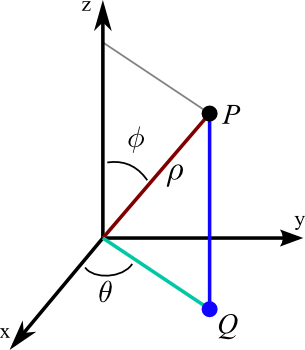# Math Insight

### Image: Spherical coordinatesIllustration of the definition of the spherical coordinates $\rho$, $\theta$, and $\phi$ of a point $P$. The coordinate $\rho$ is the distance from $P$ to the origin. If the point $Q$ is the projection of $P$ to the $xy$-plane, then $\theta$ is the angle between the positive $x$-axis and the line from the origin to $Q$. Lastly, $\phi$ is the angle between the positive $z$-axis and the line segment from the origin to $P$.

Image file: spherical_coordinates.png

Source image file: spherical_coordinates.svg
Source image type: Inkscape SVG